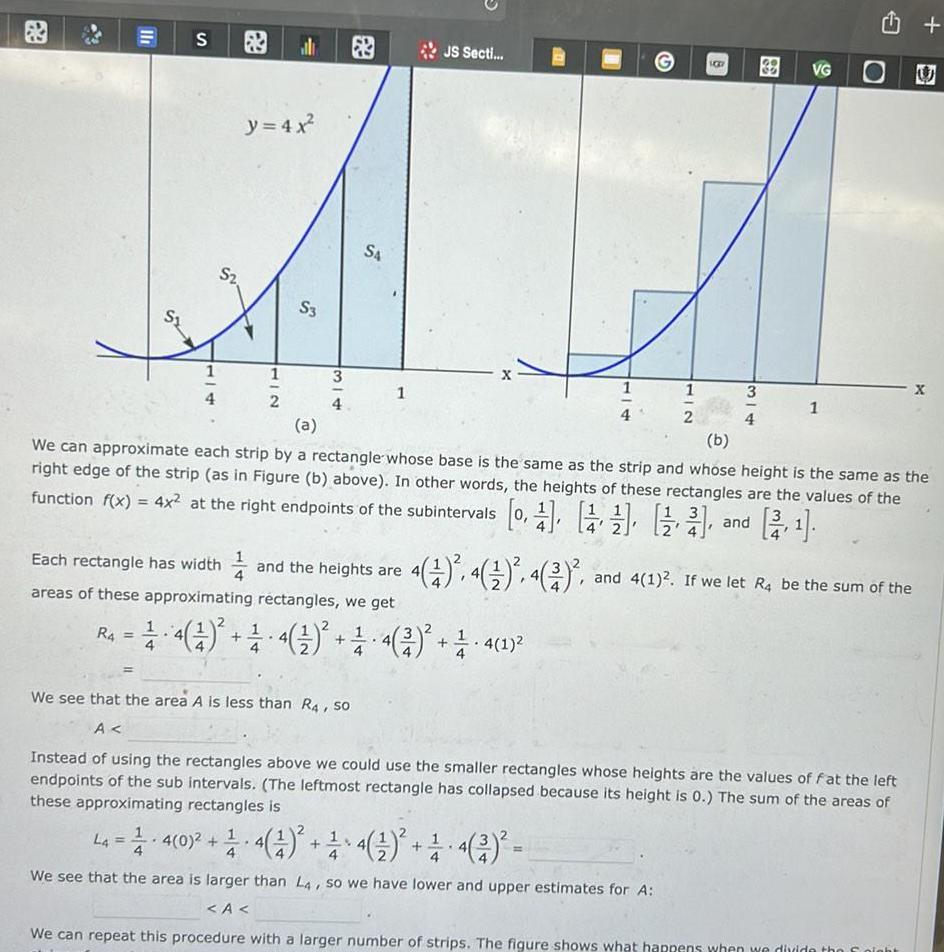Question:

# 11 S R4 1 4 S y 4x 2 HIC al 2 S3 3 4 S4 1 Each rectangle has

Last updated: 11/15/202311 S R4 1 4 S y 4x 2 HIC al 2 S3 3 4 S4 1 Each rectangle has width and the heights are areas of these approximating rectangles we get JS Secti 201 2 4 4 4 4 2 1 4 1 BB 38 3 VG 1 a b We can approximate each strip by a rectangle whose base is the same as the strip and whose height is the same as the right edge of the strip as in Figure b above In other words the heights of these rectangles are the values of the function f x 4x at the right endpoints of the subintervals 0 and 4 1 4 4 2 and 4 1 If we let R4 be the sum of the We see that the area A is less than R4 so A Instead of using the rectangles above we could use the smaller rectangles whose heights are the values of fat the left endpoints of the sub intervals The leftmost rectangle has collapsed because its height is 0 The sum of the areas of these approximating rectangles is U La 4 0 4 1 4 4 We see that the area is larger than L4 so we have lower and upper estimates for A A We can repeat this procedure with a larger number of strips The figure shows what happens when we divide the night X